×#### Thank you for registering.

One of our academic counsellors will contact you within 1 working day.

Click to Chat

1800-1023-196

+91-120-4616500

CART 0

• 0

MY CART (5)

Use Coupon: CART20 and get 20% off on all online Study Material

ITEM
DETAILS
MRP
DISCOUNT
FINAL PRICE
Total Price: Rs.

There are no items in this cart.
Continue Shopping• Complete JEE Main/Advanced Course and Test Series
• OFFERED PRICE: Rs. 15,900
• View Details

```Chapter 1: Fractions Exercise – 2.3

Question: 1

Find the reciprocal of each of the following fractions and classify them as proper, improper and whole numbers

(i) 3/7

(ii) 5/8

(iii) 9/7

(iv) 6/5

(v) 12/7

(vi) 1/8

Solution:

(i)  3/7

7/3 = improper number

(ii)  5/8

8/5 = improper number

(iii)  9/7

7/9 = proper number

(iv) 6/5

5/6 = proper number

(v) 12/7

7/12 = proper number

(vi) 1/8

8 = whole number

Question: 2

Divide:Solution:Question: 3

Divide:Solution: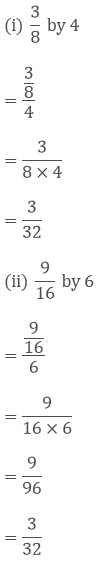Question: 4

Simplify:Solution: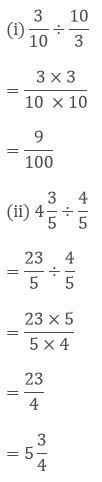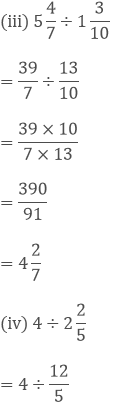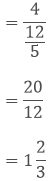Question: 5

A wire of length 12(1/2)m is cut into 10 pieces of equal length. Find the length of each piece.

Solution: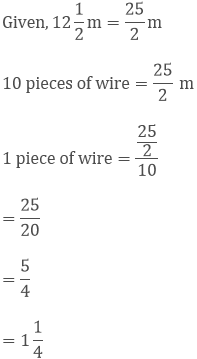Question: 6

The length of a rectangular plot of area 65(1/3) m2 is 12(1/4) m. What is the width of the plot?

Solution:

Given,

The length of a rectangular plot of area 65(1/3) m2 is 12(1/4) m.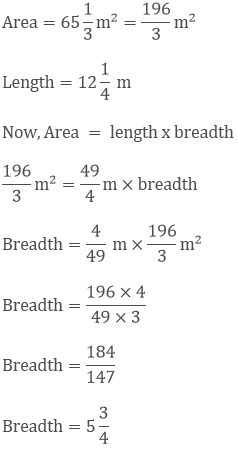Question: 7

By what number 6(2/9) be multiplied to get 4(4/9)?

Solution:

Given,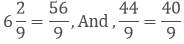Let x be the number which needs to be multiplied by 56/9,

Now,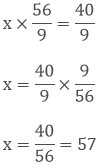Question: 8

The product of two numbers is 25(5/6). If one of the numbers is 6(2/3), find the other?

Solution:

Given,

The product of two numbers is 25(5/6). If one of the numbers is 6(2/3),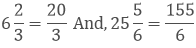Let the other number be x.Question: 9

The cost of 6(1/4) kg of apples is Rs 400. At what rate per kg are the apples being sold?

Solution:

Given,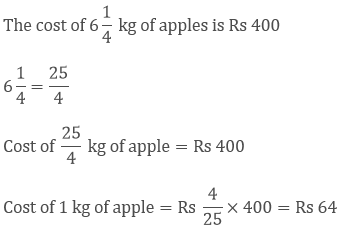Question: 10

By selling oranges at the rate of Rs 5(1/4) per orange, a fruit seller get Rs 630. How many dozens of oranges does he sell?

Solution:

Given,

By selling oranges at the rate of Rs 5(1/4) per orange, a fruit seller get Rs 630.

5(1/4) = 2(1/4)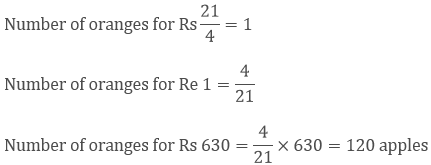12 apples =1 dozen

Therefore, 120 apples = 10 dozen

Question: 11

In mid-day meal scheme 3/10 litre of milk is given to each student of a primary school. If 30 litres of milk is distributed every-day in the school, how many students are there in the school?

Solution:

Given,

3/10  litre of milk is given to each student of a primary school.

30 litres of milk is distributed everyday in the school

Number of students given 3/10 litres of milk = 1

Number of students given 1 litre of milk = 10/3

Number of students given 30 litres of milk = 10/3 × 30 = 100 Students

Question: 12

In a charity show Rs 6496 were collected by selling some tickets. If the price of each ticket was Rs 50(3/4), how many tickets were sold?

Solution:

Given,

Rs 6496 were collected by selling some tickets. If the price of each ticket was Rs 50(3/4),```### Course Features

• 728 Video Lectures
• Revision Notes
• Previous Year Papers
• Mind Map
• Study Planner
• NCERT Solutions
• Discussion Forum
• Test paper with Video Solution